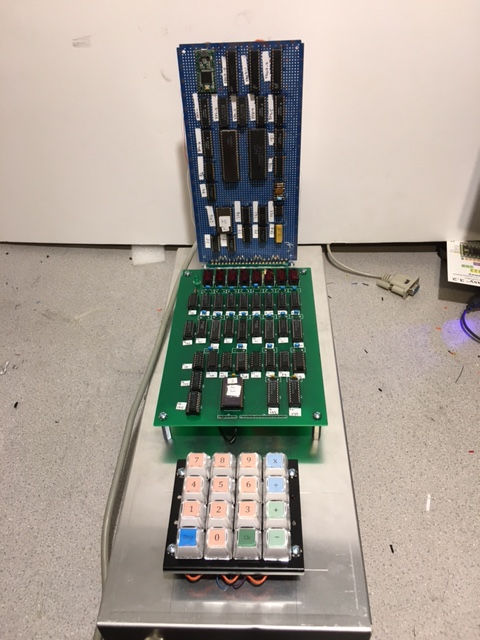top of page#### TTL Calculator

February, 2020:

I was particularly impressed with the way the Wang 360 worked - especially the way it multiplies and divides using logarithms instead of repeated addition.

I wanted to build a similar calculator 'from scratch' - using logic chips only (no calculator chips allowed).

September, 2020:

Settled on a design:

• Interface:

• 8-digit display​

• positive integers only​

• 0-9 keyboard

• two internal 8-digit registers

• "accumulator" for addition and subtraction​

• "log accumulator" for multiplication and division

• function keys:

• "+" add to accumulator and display result​

• "-" subtract from accumulator and display results

• "x" multiply into log accumulator (add log of display to log accumulator)

• "÷" divide into log accumulator (subtract log of display from log accumulator)

• "Clr" clear the accumulator

• "Disp" show the anti-log of the log accumulator (clears log accumulator)

• Hardware:

• based on AM2901 ALU and AM2910 sequence controller

• microcode ROM modeled with Teensy 3.2 (it's easier to re-program than EPROMS)

• the remainder are 74LSxx TTL chips

• mixture of custom PC board and wire-wrap

June 2021:

Finally all assembled and working:A close-up of the CPU board:Close-up of display board:

Here it is in action:

The steps are:

• key in 25+ (adds 25 to accumulator)

• key on 45+ (adds 35 to accumulator)

• accumulator is displayed: 70

• key in 33- (subtracts 33 from accumulator)

• accumulator is displayed: 37

• clear

• key in 256x (calculates log(256) and adds to log accumulator)

• key in 256x (calculates log(256) and adds to log accumulator)

• Disp - displays anti-log of log accumulator (65536)

• clear

• then calculate 7894 x 562 to give 4436422 (real answer is 4436428 - close!)

bottom of page